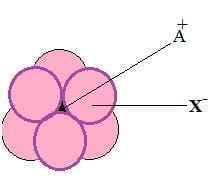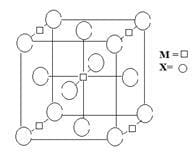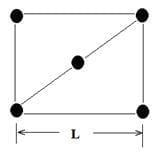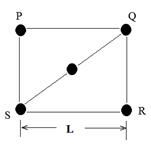# Solved Examples: Solid State Notes | Study Mock Test Series for JEE Main & Advanced 2022 - JEE

## JEE: Solved Examples: Solid State Notes | Study Mock Test Series for JEE Main & Advanced 2022 - JEE

The document Solved Examples: Solid State Notes | Study Mock Test Series for JEE Main & Advanced 2022 - JEE is a part of the JEE Course Mock Test Series for JEE Main & Advanced 2022.
All you need of JEE at this link: JEE

Question 1: The arrangement of X ions around A+ ion in solid AX is given in the figure (not drawn to scale). If the radius of X– is 250 pm, the radius of A+ is    (IIT JEE 2013)(a) 104 pm
(b) 125 pm
(c) 183 pm
(d) 57 pm
Solution:
A+ is present in the octahedral void of X-.
For octahedral Void
r+/r- = 0.414
or
r+ = 0.414 r- = 0.414 × 250 pm ≈104 pm
Hence, the correct option is a.

Question 2: A compound MpXq has cubic close packing (ccp) arrangement of X. Its unit cell structure is shown below.    (IIT JEE-2012)The empirical formula of the compound is
(a) MX
(b) MX2
(c) M2X
(d) M5X14
Solution:
X- ions are present at the corners and  face centers:
Number of x- ions per lattice = 1/8 (Number of ions present at corners of cube) + ½ (Number of ions present at face centers) = 1/8(8) + ½(6) = 4
M+ ions are present at the four edge centers and at body center. corners and on face centers:
Number of M+ ions per lattice = 1/4(Number of ions present at edge centers of cube) + (Number of ions present at body center) = 1/4(4) + 1 = 2
Now: X: M = 4:2 = 2:1
So, the formula should be MX2
Hence, the correct option is b.

Question 3: The packing efficiency of the two-dimensional square unit cell shown below is   (IIT JEE-2010)(a) 39.27%
(b) 68.02%
(c) 74.05%
(d) 78.54%
Solution:Total number of the atoms per unit cell = ¼(4) +1 = 2
Let, Radious of the atom = r
SR = QR =PQ = SP =L
&
QS = 4r
Now, in triangle SRP,
SR+ QR2 =SQ2
Or
L2+L2 =(4r)2
r = L/(2√2 )
Or
Packing Efficiency = [(2× Volume of one atom)/(Total volume of the unit cell)]×100
=100 × {2π[L/(2√2 )]2 }/L2
= (π/8 )×100 = 78.54%
Hence, the correct option is D.

The document Solved Examples: Solid State Notes | Study Mock Test Series for JEE Main & Advanced 2022 - JEE is a part of the JEE Course Mock Test Series for JEE Main & Advanced 2022.
All you need of JEE at this link: JEEUse Code STAYHOME200 and get INR 200 additional OFF

## Mock Test Series for JEE Main & Advanced 2022

2 videos|258 docs|160 tests

Track your progress, build streaks, highlight & save important lessons and more!

,

,

,

,

,

,

,

,

,

,

,

,

,

,

,

,

,

,

,

,

,

;